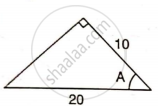# Find Angle 'A' If : - Mathematics

Sum

Find angle 'A' if :#### Solution

From the figure we have

cos A = (10)/(20)

cos A = (1)/(2)

cos A = cos 60°

A = 60°

Concept: Solution of Right Triangles
Is there an error in this question or solution?

#### APPEARS IN

Selina Concise Mathematics Class 9 ICSE
Chapter 24 Solution of Right Triangles [Simple 2-D Problems Involving One Right-angled Triangle]
Exercise 24 | Q 2.1 | Page 303